Open in App
Not now

# Forces Between Multiple Charges

• Last Updated : 13 Feb, 2022

When our synthetic clothing or sweater is removed from our bodies, especially in dry weather, a spark or crackling sound appears. With females’ clothing like a polyester saree, this is almost unavoidable. Lightning, in the sky during thunderstorms, is another case of electric discharge. It is an electric shock always felt while opening a car door or grabbing the iron bar of a bus after sliding out of our seats. The cause of these sensations is the discharge of electric charges that have collected as a result of rubbing insulating surfaces. This is due to static electricity generation. Anything that does not have movement or change with time is referred to as static. The study of forces, fields, and potentials coming from static charges is known as electrostatics.

### Coulomb’s Law

Coulomb’s law is a mathematical formula that describes the force between two point charges. When the size of charged bodies is substantially smaller than the separation between them, then the size is not considered or can be ignored. The charged bodies can be considered as point charges. Coulomb studied the force between two point charges and found that it is inversely proportional to the square of the distance between them, directly proportional to the product of their magnitudes, and acting in a line that connects them.

Expression for Coulomb’s Law

The amount of the force (F) between two point charges q1 and q2 separated by a distance r in a vacuum is given byWhere F is the force between two point charges, q1 and q2 are the point charge, r is the distance between the point charge and k is proportionality constant. For subsequent simplicity, the constant k in the above expression is commonly written asHere,is known as the permittivity of free space. The value ofin SI units isCoulomb’s Law in Vector Form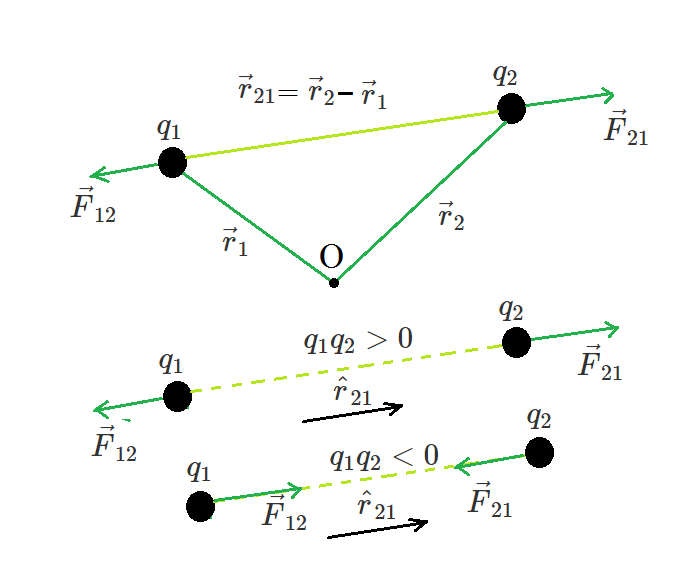Geometry and Forces between charges

Coulomb’s law is better written in vector notation because force is a vector quantity. Charges q1 and q2 have location vectors r1 and r2, respectively. F12 denotes force on q1 owing to q2 and F21 denotes force on q2 owing to q1. For convenience, the two-point charges q1 and q2 have been numbered 1 and 2, respectively, and the vector leading from 1 to 2 has been designated by r21.Similarly, the vector leading from 2 to 1 is denoted by r12,r21 and r12 are the magnitudes of the vectorsand, respectively and magnitude r12 is equal to r21. A unit vector along the vector specifies the vector’s direction. The unit vectors are used to denote the direction from 1 to 2 (or 2 to 1). The unit vectors define as,Similarly,Coulomb’s force law between two point charges q1 and q2 located at vector r1 and r2 is then expressed as### Force Between Multiple Charges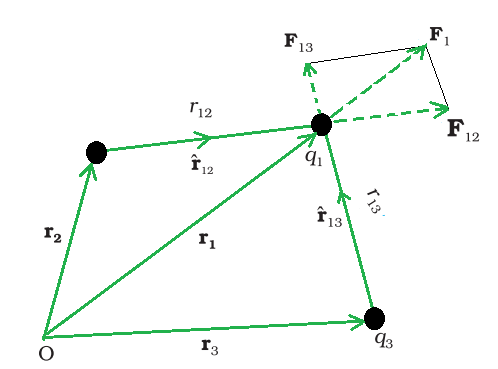A system of three charges.

Consider a system in a vacuum with n motionless that is stationary charges q1, q2, and q3. It has been proven experimentally that the vector sum of all the forces on a charge due to a number of other charges, taken one at a time, is the vector sum of all the forces on that charge owing to the other charges. Due to the presence of other charges, the separate forces remain unaffected. This is known as the superposition principle.

The force on one charge, say q1, due to two other charges, q2 and q3, may be determined by conducting a vector addition of the forces due to each of these charges. As a result, if F12 denotes the force exerted on q1 as a result of q2,Similarly, F13 denotes the force exerted on q1 as a result of q3, which again is the Coulomb force on q1 due to q3 even though other charge q2 is present. Thus, the total force F1 on q1 due to the two charges q2 and q3 can be expressed as,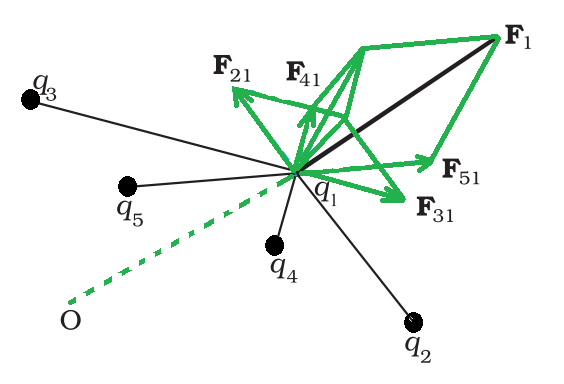A system of multiple charges.

The above force calculation can be applied to a system with more than three charges. The principle of superposition states that in a system of charges q1, q2…….qn, the force on q1 owing to q2 is the same as Coulomb’s law, i.e., it is unaffected by the presence of other charges q3, q4,…, qn. The vector sum of the forces F12, F13,…, F1n on the charge q1 owing to all other charges gives the overall force F1 can be written asThe vector sum is calculated by using the parallelogram law of vector addition. Coulomb’s law and the superposition principle are the foundations of electrostatics.

Key Points on Coulomb’s Law

• The above expression holds true regardless of whether q1 and q2 are positive or negative. F21 is toward, which repulsive force, as it should be for like charges that are if q1 and q2 are of the same sign (either both positive or both negative). When the signs of q1 and q2 are opposite or dislike charge, F21 is toward, that is towardwhich shows attraction, as expected for dissimilar charges. As a result, we don’t need to construct separate equations for like and unlike charges. Both instances are handled correctly by the above expression for Coulomb’s force law.
• The above expression for Coulomb’s force law can be used to calculate the force F12 on charge q1 due to charge q2 by simply swapping 1 and 2 as,Coulomb’s law therefore agrees with Newton’s third law.

• In a vacuum, Coulomb’s law expression determines the force between two charges q1 and q2. If the charges are deposited in matter or there is matter in the intervening area, the situation becomes more complicated due to the presence of charged matter constituents.
• Two identical conductors with charges q1 and q2 are brought into contact and subsequently separated, resulting in each conductor having a charge equal to (q1+q2)/2. Each charge will be equal to (q1-q2)/2 if the charges are q1 and –q2.

Electric Charge

The word “electricity” comes from the Greek word “electron,” which means “amber”. The magnetic and electric forces present in materials, atoms, and molecules affect their properties. The term “electric charge” refers to just two types of entities. According to the results of an experiment, there are two types of electrification:

• Like charges repel one another and
• Unlike charges attract each other

The polarity of charge is the feature that distinguishes these two types of charges.

Conductors and Insulators

When an experiment on electric charges caused by frictional electricity was carried out, it was discovered that conductors aid in the flow of electric charge, whereas insulators do not. Metal, Earth, and Human Bodies are all conductors, but porcelain, nylon, and wood are all insulators, offering high resistance to the flow of electricity through them.

Properties of Electric charge

There are three basic qualities of an electric charge:

• Quantization: The total charge of a body indicates the integral multiple of a basic quantum of charge.
• Additive: This electric charge property reflects a body’s overall charge as the algebraic sum of all singular charges acting on the system.
• Conservation: The entire charge of a system remains unchanged throughout time, according to conservation. In other words, when objects become charged as a result of friction, the charge is transferred from one object to another. There is no way to create or eliminate charges.

Properties of Electric Field Lines

The following are some of the general characteristics of field lines:

• In a charge-free zone, field lines show a continuous curve with no breaks.
• Electric Field Lines never cross each other.
• These electric field lines begin with a positive charge and conclude with a negative charge.
• Electrostatic field lines do not form any closed loops.

### Sample Problems

Problem 1: A positive charge of 6×10-6 C is 0.040 m from the second positive charge of 4×10-6 C. Calculate the force between the charges.

Solution:

Given,

A positive charge q1 is 6×10-6 C.

The second positive charge q2 is 4×10-6 C.

The distance between the charges r  is 0.040 m.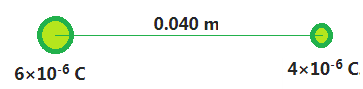Substitute the values in the above expression,Problem 2: Four equal charges q are kept on corners of a square of the side ‘r’, find the net force on one of the charges.

Solution:

Given,

Four equal charges ‘q’ and the distance between them is r.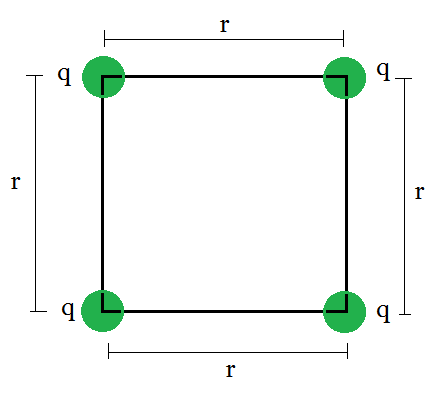The individual repulsive forces on the corner charge q and make a vector diagram and note down the forces, which as: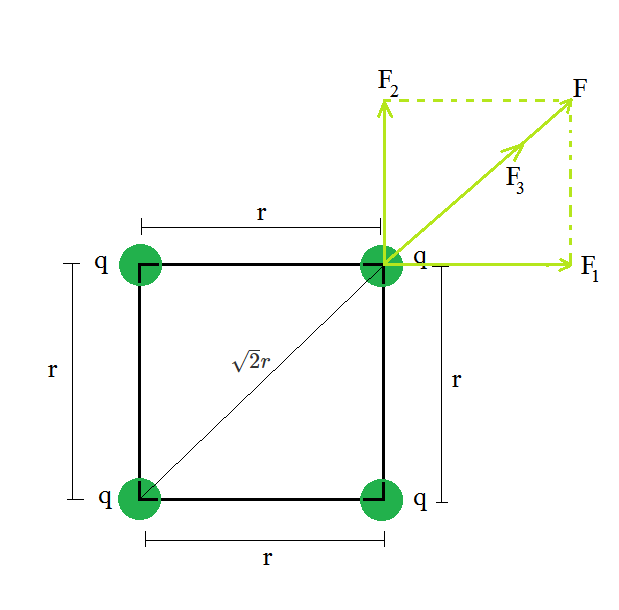The force due to the Left top charge on the right top charge is F1 and can be expressed as,

F1 = (kq2)/r2

The force due to the right bottom charge on the right top charge is F2 and can be expressed as,

F2 = (kq2)/r2

The force due to the Left bottom charge on the right top charge is F3 and can be expressed as,The resultant force on the right top charge can be written as,Here cos 90o is equal to 0.

Substitute the value in the above expression.Problem 3: Charges of magnitude 100 microcoulomb each are located in vacuum at the corners A, B, and C of an equilateral triangle measuring 4 meters on each side. If the charge at A and C are positive and the charge B negative, what is the magnitude and direction of the total force on the charge at C?

Solution: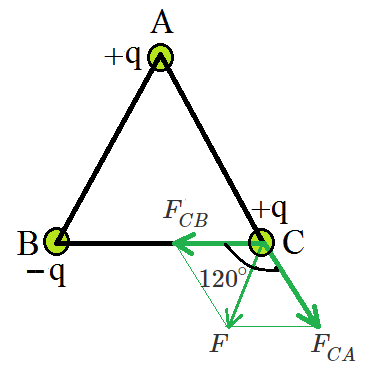The Force FCA is applied toward AC and the expression for the FCA is expressed asSubstitute the values in the above expression,The Force FCB is applied toward CB and the expression for the FCB is expressed asSubstitute the values in the above expression,Therefore, the two forces are equal in magnitude but in different directions. The angle between them is 120º. The resultant force F is given by,Problem 4: Compare the nature of electrostatic and gravitational forces.

Solution:

Between two huge masses, a gravitational force acts. However, an electrostatic force is activated when two charged bodies come into contact.

Similarities:

1. These two forces are central forces.
2. Follow the law of inverse squares.
3. They’re both long-range forces.
4. Both forces are naturally conservative.

Dissimilarities:

1. In nature, electrostatic force can be both attractive and repellent. In nature, gravitational force can only be attractive.
2. The material medium between two charges affects the electric force between them. The material medium between huge bodies has little effect on gravitational force.
3. Electric forces are extremely powerful approximately 10 38 times stronger) than gravitational forces.

Problem 5: Why does Coulomb’s force act between two charges only in the line joining their centers?

Solution:

Because of the fundamental features of electrical charge, this is the case. Charges that are similar repel each other. Charges that are diametrically opposed attract each other.

The force of attraction or repulsion between two charges will be directed in the direction so that the force does the least amount of work. As a result of this requirement, the action is directed along the straight line connecting the two charges, which is the shortest distance between them.

My Personal Notes arrow_drop_up